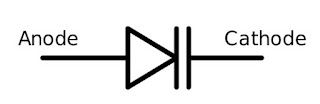## Search This Blog

### Varactor diode symbol

The symbol of the varactor diode is shown in the figure is given below. The varactor diode symbol consists of with capacitor symbol at the one end of the diode that represents the variable capacitor type characteristics of the varactor diodes to shown in figure.

In general, it looks like or similar to that a normal PN junction diode in which one terminal is termed as the cathode and the other terminal is termed as an anode. Here the varactor diode consists of two lines at one end cathode end of normal diode that indicates the capacitor symbol. The one end of a symbol consists of the diode and their other end has two parallel lines.Varactor Diode Symbol

The symbol of the varactor diode is shown in the figure is given below. The varactor diode symbol consists of with capacitor symbol at the one end of the diode that represents the variable capacitor type characteristics of the varactor diodes to shown in figure.

In general, it looks like or similar to that a normal PN junction diode in which one terminal is termed as the cathode and the other terminal is termed as an anode. Here the varactor diode consists of two lines at one end cathode end of normal diode that indicates the capacitor symbol. The one end of a symbol consists of the diode and their other end has two parallel lines.Varactor Diode Symbol